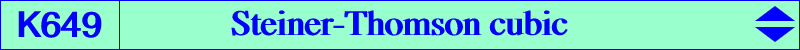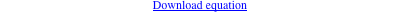too complicated to be written here. Click on the link to download a text file.X(5), X(20), X(69), X(381) cusps of the Steiner deltoid of ABC vertices of the cevian triangle of X(69) infinite points of the Thomson cubicThe cubics passing through the cusps of the Steiner deltoid of ABC, the vertices of the cevian triangle of X(69), the centers X(5), X(20) form a pencil which contains one circum-cubic namely the Steiner-McCay cubic K071. Each cubic of the pencil meets the Euler line at a third point X and then also contains the infinite points of the isogonal pK with pivot the reflection of X about X(5). When X = X(381), the cubic is K649 and the pK is the Thomson cubic. The cubic and the pK meet at six other finite points which lie on a same rectangular hyperbola and all these hyperbolas belong to a same pencil. The pencil above contains K648, K649, K650, K651, K652.